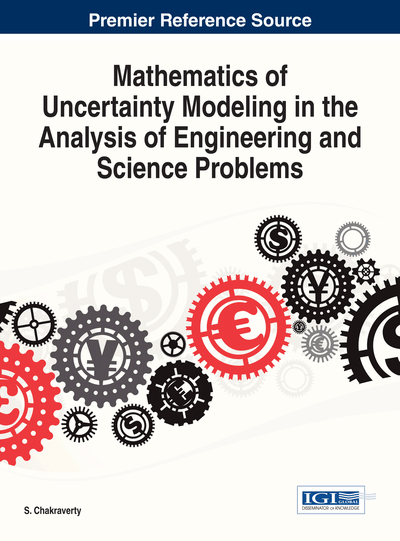# Source and m-Source Distances of Fuzzy Numbers and their Properties

DOI: 10.4018/978-1-4666-4991-0.ch005

## Abstract

In many applications of fuzzy logic and fuzzy mathematics we need (or it is better) to work with the same fuzzy numbers. In this chapter we present source distance and source distance between two fuzzy numbers. Also some properties of parametric -degree polynomial approximation operator of fuzzy numbers. Numerical examples are solved related to the present analysis.
Chapter Preview
Top

## 1. Introduction

In some applications of fuzzy logic, we need to compare two fuzzy numbers. In order to make the comparisn easier we try to find some quantities related to the fuzzy numbers to use in comparison.

In (Abbasbandy & Amirfakhrian, 2006(1)) and (Abbasbandy & Amirfakhrian, 2006(2)) two nearest trapezoidal form of a fuzzy number by using a parametric form are introduced. Abbasbandy and Asadi (2004) have introduced the nearest trapezoidal fuzzy number to a fuzzy quantity by an optimization problem. Delgado, Vila and Voxman (1998), introduced a canonical representation of fuzzy numbers. A parameterized approximation of a fuzzy number with a minimum variance weighting function presented by Liua & Lin, (2007). A weighted triangular approximation of a fuzzy number is introduced in (Zeng & Li, 2007). Yeh (2007) and (2008) presented trapezoidal and triangular approximations. In (Ban, 2008) and (Ban, 2009) two types of approximation of fuzzy numbers are presented. In all of the mentioned literatures the authors tried to approximate a fuzzy number by a simpler one.

Also there are some distances defined by authors to compare fuzzy numbers. Voxman (1998), Yao & Wu, (2000), Ma, Kandel & Friedman, (2000) and Ma, Kandel & Friedman, (2002) introduced some distances which are examples of these comparisons.

Obviously, if we use a defuzzification rule which replaces a fuzzy set by a single number, we generally loose too many important information. Also, an interval approximation is considered for fuzzy numbers in (Grzegorzewski, 2002), where a fuzzy computation problem is converted into interval arithmetic problem. But, in this case, we loose the fuzzy central concept. Even in some works such as (Abbasbandy & Asadi, 2004), (Ma, Kandel & Friedman, 2000) and (Ma, Kandel & Friedman, 2002) and (Zeng & Li, 2007), authors solve an optimization problem to obtain the nearest triangular or trapezoidal fuzzy number which is related to an arbitrary fuzzy number, however in these cases there is not any guarantee to have the same modal value (or interval). But by parametric polynomial approximation we are able to approximate many fuzzy numbers in a good manner.

Recently the problem of finding the nearest parametric approximation of a fuzzy number with respect to the average Euclidean distance is completely solved in (Ban, 2009). Ban pointed out some wrongs and inadvertences of some recent papers, and then corrected the results in (Ban, 2008). A parametric fuzzy approximation method based on the decision makers strategy as an extension of trapezoidal approximation of a fuzzy number offered in (Nasibov & Peker, 2008). An improvement of the nearest trapezoidal approximation operator preserving the expected interval, which is proposed by Grzegorzewski and Mrówka is studied in (Grzegorzewski &, 2008). There are some trapezoidal approximation operators introduced in (Grzegorzewski &, 2007), (Grzegorzewski &, 2008) and (Yeh, 2007).

## Complete Chapter List

Search this Book:
Reset